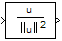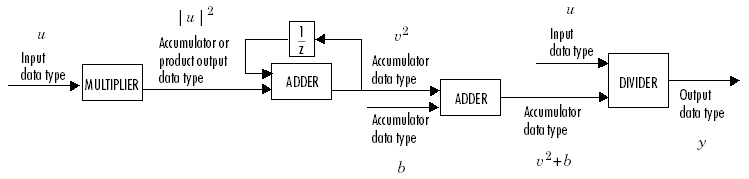# Normalization

Perform vector normalization along rows, columns, or specified dimension

## Library

Math Functions / Math Operations

`dspmathops`

•## Description

The Normalization block independently normalizes each row, column, or vector of the specified dimension of the input. The block accepts both fixed- and floating-point signals in the squared 2-norm mode, but only floating-point signals in the 2-norm mode. The output always has the same dimensions as the input.

This block treats an arbitrarily dimensioned input U as a collection of vectors oriented along the specified dimension. The block normalizes these vectors by either their norm or the square of their norm.

For example, consider a 3-dimensional input U(i,j,k) and assume that you want to normalize along the second dimension. First, define the 2-dimensional intermediate quantity V(i,k) such that each element of V is the norm of one of the vectors in U:

`$V\left(i,k\right)={\left(\sum _{j=1}^{J}{U}^{2}\left(i,j,k\right)\right)}^{1/2}$`

Given V, the output of the block Y(i, j,k) in 2-norm mode is

`$Y\left(i,j,k\right)=\frac{U\left(i,j,k\right)}{V\left(i,k\right)+b}$`

In squared 2-norm mode, the block output is

`$Y\left(i,j,k\right)=\frac{U\left(i,j,k\right)}{V{\left(i,k\right)}^{2}+b}$`

The normalization bias, b, is typically chosen to be a small positive constant (for example, 1e-10) that prevents potential division by zero.

### Fixed-Point Data Types

The following diagram shows the data types used within the Normalization block for fixed-point signals (squared 2-norm mode only).The output of the multiplier is in the product output data type when the input is real. When the input is complex, the result of the multiplication is in the accumulator data type. For details on the complex multiplication performed, see Multiplication Data Types. You can set the accumulator, product output, and output data types in the block dialog as discussed in Parameters.

## Examples

See Zero Algorithmic Delay in the DSP System Toolbox™ User's Guide for an example.

## Parameters

Main Tab

Norm

Specify the type of normalization to perform, `2-norm` or ```Squared 2-norm```. `2-norm` mode supports floating-point signals only. `Squared 2-norm` supports both fixed-point and floating-point signals.

Normalization bias

Specify the real value b to be added in the denominator to avoid division by zero. Tunable (Simulink).

Normalize over

Specify whether to normalize along rows, columns, or the dimension specified in the Dimension parameter.

Dimension

Specify the one-based value of the dimension over which to normalize. The value of this parameter cannot exceed the number of dimensions in the input signal. This parameter is only visible if ```Specified dimension``` is selected for the Normalize over parameter.

Data Types Tab

Note

The parameters on this pane are only applicable to fixed-point signals when the block is in squared 2-norm mode. See Fixed-Point Data Types for a diagram of how the product output, accumulator, and output data types are used in this case.

Rounding mode

Specify the rounding mode for fixed-point operations as one of the following:

• `Floor`

• `Ceiling`

• `Convergent`

• `Nearest`

• `Round`

• `Simplest`

• `Zero`

For more details, see rounding mode.

Saturate on integer overflow

When you select this parameter, the block saturates the result of its fixed-point operation. When you clear this parameter, the block wraps the result of its fixed-point operation. For details on `saturate` and `wrap`, see overflow mode for fixed-point operations.

Product output

Specify the product output data type. See Fixed-Point Data Types and Multiplication Data Types for illustrations depicting the use of the product output data type in this block. You can set it to:

• A rule that inherits a data type, for example, `Inherit: Same as input`

• An expression that evaluates to a valid data type, for example, `fixdt([],16,0)`

Click the buttonto display the Data Type Assistant, which helps you set the Product output parameter.

Accumulator

Specify the accumulator data type. See Fixed-Point Data Types for illustrations depicting the use of the accumulator data type in this block. You can set this parameter to:

• A rule that inherits a data type, for example, ```Inherit: Same as product output```

• A rule that inherits a data type, for example, `Inherit: Same as input`

• An expression that evaluates to a valid data type, for example, `fixdt([],16,0)`

Click the buttonto display the Data Type Assistant, which helps you set the Accumulator parameter.

Output

Specify the output data type. See Fixed-Point Data Types for illustrations depicting the use of the output data type in this block. You can set it to:

• A rule that inherits a data type, for example, ```Inherit: Same as product output```

• A rule that inherits a data type, for example, `Inherit: Same as input`

• A rule that inherits a data type, for example, `Inherit: Same as accumulator`

• An expression that evaluates to a valid data type, for example, `fixdt([],16,0)`

Click the buttonto display the Data Type Assistant, which helps you set the Output parameter.

Output Minimum

Specify the minimum value that the block should output. The default value is `[]` (unspecified). Simulink® software uses this value to perform:

• Simulation range checking (see Specify Signal Ranges (Simulink))

• Automatic scaling of fixed-point data types

Output Maximum

Specify the maximum value that the block should output. The default value is `[]` (unspecified). Simulink software uses this value to perform:

• Simulation range checking (see Specify Signal Ranges (Simulink))

• Automatic scaling of fixed-point data types

Lock data type settings against changes by the fixed-point tools

Select this parameter to prevent the fixed-point tools from overriding the data types you specify on the block mask.

## Supported Data Types

PortSupported Data Types

Input

• Double-precision floating point

• Single-precision floating point

• Fixed point (signed and unsigned)

• 8-, 16-, and 32-bit signed integers

• 8-, 16-, and 32-bit unsigned integers

Output

• Double-precision floating point

• Single-precision floating point

• Fixed point (signed and unsigned)

• 8-, 16-, and 32-bit signed integers

• 8-, 16-, and 32-bit unsigned integers

## Extended Capabilities

### Blocks

Introduced before R2006a

## SupportGet trial now